# errorbar

Line plot with error bars

•## Syntax

``errorbar(y,err)``
``errorbar(x,y,err)``
``errorbar(x,y,neg,pos)``
``errorbar(___,ornt)``
``errorbar(x,y,yneg,ypos,xneg,xpos)``
``errorbar(___,LineSpec)``
``errorbar(___,Name,Value)``
``errorbar(ax,___)``
``e = errorbar(___)``

## Description

````errorbar(y,err)` creates a line plot of the data in `y` and draws a vertical error bar at each data point. The values in `err` determine the lengths of each error bar above and below the data points, so the total error bar lengths are double the `err` values.```

example

````errorbar(x,y,err)` plots `y` versus `x` and draws a vertical error bar at each data point.```
````errorbar(x,y,neg,pos)` draws a vertical error bar at each data point, where `neg` determines the length below the data point and `pos` determines the length above the data point, respectively.```

example

````errorbar(___,ornt)` sets the orientation of the error bars. Specify `ornt` as `"horizontal"` for horizontal error bars or `"both"` for both horizontal and vertical error bars. The default for `ornt` is `"vertical"`, which draws vertical error bars. Use this option after any of the previous input argument combinations.```

example

````errorbar(x,y,yneg,ypos,xneg,xpos)` plots `y` versus `x` and draws both horizontal and vertical error bars. `yneg` and `ypos` set the lower and upper lengths of the vertical error bars, respectively. Similarly, `xneg` and `xpos` set the left and right lengths of the horizontal error bars.```

example

````errorbar(___,LineSpec)` sets the line style, marker symbol, and color. For example, `"--ro"` plots a dashed, red line with circle markers. The line style affects only the line and not the error bars.```

example

````errorbar(___,Name,Value)` modifies the appearance of the line and error bars using one or more name-value pair arguments. For example, `"CapSize",10` sets the lengths of the caps at the end of each error bar to 10 points.```
````errorbar(ax,___)` creates the plot in the axes specified by `ax` instead of in the current axes. Specify the axes as the first input argument.```

example

````e = errorbar(___)` returns one `ErrorBar` object for each plotted line. Use `e` to modify properties of a specific `ErrorBar` object after it is created. For a list of properties, see ErrorBar Properties.```

## Examples

collapse all

Create vectors `x` and `y`. Plot `y` versus `x`. At each data point, display vertical error bars that are equal in length.

```x = 1:10:100; y = [20 30 45 40 60 65 80 75 95 90]; err = 8*ones(size(y)); errorbar(x,y,err)```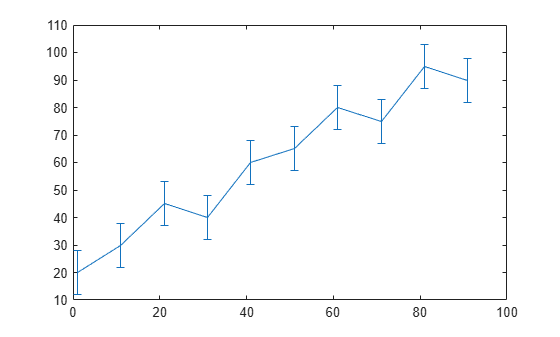Create a line plot with error bars at each data point. Vary the lengths of the error bars.

```x = 1:10:100; y = [20 30 45 40 60 65 80 75 95 90]; err = [5 8 2 9 3 3 8 3 9 3]; errorbar(x,y,err)```Create a line plot with horizontal error bars at each data point.

```x = 1:10:100; y = [20 30 45 40 60 65 80 75 95 90]; err = [1 3 5 3 5 3 6 4 3 3]; errorbar(x,y,err,'horizontal')```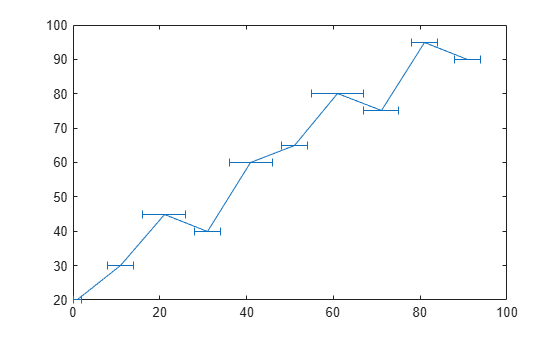Create a line plot with both vertical and horizontal error bars at each data point.

```x = 1:10:100; y = [20 30 45 40 60 65 80 75 95 90]; err = [4 3 5 3 5 3 6 4 3 3]; errorbar(x,y,err,'both')```Plot vectors `y` versus `x`. At each data point, display a circle marker with both vertical and horizontal error bars. Do not display the line that connects the data points by omitting the line style option for the `linespec` input argument.

```x = 1:10:100; y = [20 30 45 40 60 65 80 75 95 90]; err = [4 3 5 3 5 3 6 4 3 3]; errorbar(x,y,err,"both","o")```Alternatvely, omit the markers and plot the error bars by themselves. To do this, specify the `LineStyle` name-value argument as `"none"`.

`errorbar(x,y,err,"both","LineStyle","none")`Display both vertical and horizontal error bars at each data point. Control the lower and upper lengths of the vertical error bars using the `yneg` and `ypos` input argument options, respectively. Control the left and right lengths of the horizontal error bars using the `xneg` and `xpos` input argument options, respectively.

```x = 1:10:100; y = [20 30 45 40 60 65 80 75 95 90]; yneg = [1 3 5 3 5 3 6 4 3 3]; ypos = [2 5 3 5 2 5 2 2 5 5]; xneg = [1 3 5 3 5 3 6 4 3 3]; xpos = [2 5 3 5 2 5 2 2 5 5]; errorbar(x,y,yneg,ypos,xneg,xpos,'o')```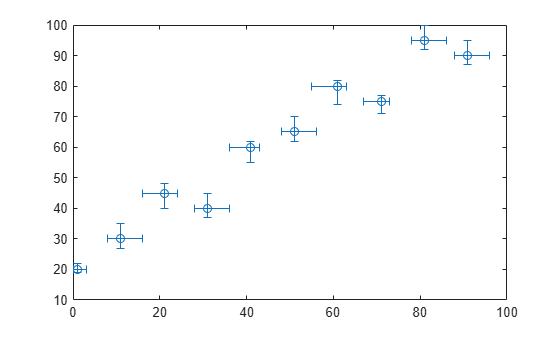Create a plot of datetime values with error bars in duration units.

```x = 1:13; y = datetime(2018,5,1,1:13,0,0); err = hours(rand(13,1)); errorbar(x,y,err)```Create a line plot with error bars. At each data point, display a marker. Change the appearance of the marker using name-value arguments. Use `MarkerSize` to specify the marker size in points. Use `MarkerEdgeColor` and `MarkerFaceColor` to specify the marker outline and fill colors, respectively. You can specify colors by name, such as "blue`"`, as RGB triplets, or hexadecimal color codes.

```x = linspace(0,10,15); y = sin(x/2); err = 0.3*ones(size(y)); errorbar(x,y,err,"-s","MarkerSize",10,... "MarkerEdgeColor","blue","MarkerFaceColor",[0.65 0.85 0.90])```Control the size of the caps at the end of each error bar by setting the `CapSize` property to a positive value in points.

```x = linspace(0,2,15); y = exp(x); err = 0.3*ones(size(y)); e = errorbar(x,y,err,'CapSize',18);```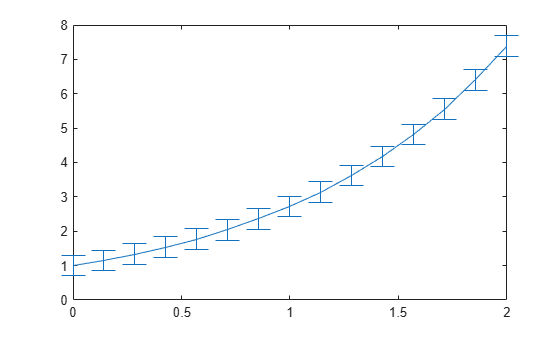To remove the caps, set the cap size to zero. Then add a margin of padding around the inside of the plot box by calling the `axis padded` command. Adding this margin keeps the error bars from overlapping with the plot box.

```e.CapSize = 0; axis padded```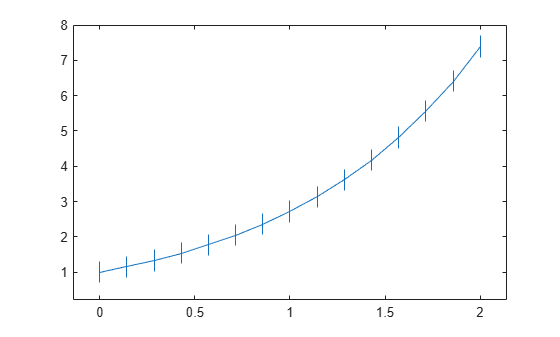Create a line plot with error bars. Assign the errorbar object to the variable `e`.

```x = linspace(0,10,10); y = sin(x/2); err = 0.3*ones(size(y)); e = errorbar(x,y,err)```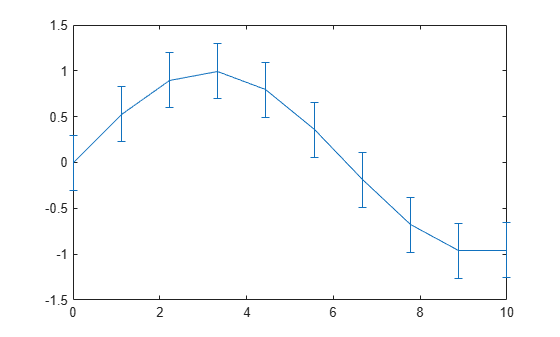```e = ErrorBar with properties: Color: [0 0.4470 0.7410] LineStyle: '-' LineWidth: 0.5000 Marker: 'none' XData: [0 1.1111 2.2222 3.3333 4.4444 5.5556 6.6667 7.7778 ... ] YData: [0 0.5274 0.8962 0.9954 0.7952 0.3558 -0.1906 ... ] XNegativeDelta: [1x0 double] XPositiveDelta: [1x0 double] YNegativeDelta: [0.3000 0.3000 0.3000 0.3000 0.3000 0.3000 0.3000 ... ] YPositiveDelta: [0.3000 0.3000 0.3000 0.3000 0.3000 0.3000 0.3000 ... ] Show all properties ```

Use `e` to access properties of the errorbar object after it is created.

```e.Marker = '*'; e.MarkerSize = 10; e.Color = 'red'; e.CapSize = 15;```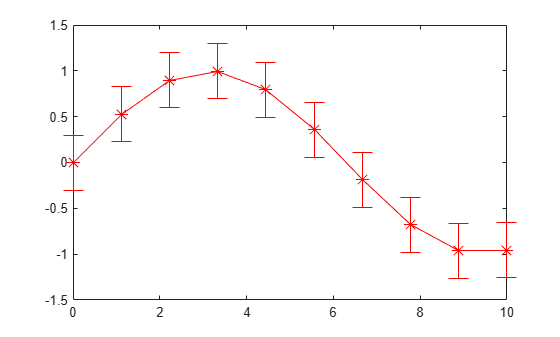## Input Arguments

collapse all

y-coordinates, specified as a vector or matrix. The size and shape of `y` depend on the size and shape of your data and the type of plot you want to make. This table describes the most common types of plots you can create.

Type of PlotCoordinates and Error Bar Lengths
One line with error bars

Specify all coordinates and error bar lengths as any combination of row and column vectors of the same length. For example, plot one line with error bars. Adjust the x-axis limits with the `xlim` function to prevent any overlap between the error bars and the plot box.

```y = 1:5; err = [0.3 0.1 0.3 0.1 0.3]; errorbar(y,err) xlim([0.9 5.1])```

Optionally specify `x` as a vector of the same length as `y`.

```x = [0; 1; 2; 3; 4]; y = 1:5; err = [0.3 0.1 0.3 0.1 0.3]; errorbar(x,y,err) xlim([-0.1 4.1])```

Multiple lines with error bars

Specify one or more of the coordinate inputs or error bar lengths as matrices. All matrices must be the same size and orientation. If any inputs are specified as vectors, they must have the same number of elements, and they must have the same length as one of the dimensions of the matrices.

MATLAB® plots one line for each column in the matrices in these situations:

• When all the coordinates and the error bar lengths are matrices of the same size and orientation

• When all the inputs specified as vectors are the same length as the columns of the matrices

For example, plot five lines that each have two error bars. Adjust the x-axis limits with the `xlim` function to prevent any overlap between the error bars and the plot box.

```y = [1 2 3 4 5; 2 3 4 5 6]; err = [0.2 0.1 0.3 0.1 0.2; 0.1 0.3 0.4 0.3 0.1]; errorbar(y,err) xlim([0.95 2.05])```

Using the same `err` values from the preceding code, specify `x` as a 2-by-5 matrix and `y` as a five-element vector. The resulting plot has two lines that each have five error bars. The y-coordinates are shared between the two lines.

```x = [0 1 2 3 4; 10 11 12 13 14]; y = [1 2 3 4 5]; err = [0.2 0.1 0.3 0.1 0.2; 0.1 0.3 0.4 0.3 0.1]; errorbar(x,y,err) xlim([-0.5 14.5])```

Data Types: `single` | `double` | `int8` | `int16` | `int32` | `int64` | `uint8` | `uint16` | `uint32` | `uint64` | `logical` | `categorical` | `datetime` | `duration`

x-coordinates, specified as a vector or matrix. The size and shape of `x` depend on the size and shape of your data and the type of plot you want to make. This table describes the most common types of plots you can create.

Type of PlotCoordinates and Error Bar Lengths
One line with error bars

Specify all coordinates and error bar lengths as any combination of row and column vectors of the same length. For example, plot one line with error bars. Adjust the x-axis limits with the `xlim` function to prevent any overlap between the error bars and the plot box.

```x = 0:4; y = [1; 2; 3; 4; 5]; err = [0.2 0.1 0.3 0.1 0.2]; errorbar(x,y,err) xlim([-0.1 4.1])```

Multiple lines with error bars

Specify one or more of the coordinate inputs or error bar lengths as matrices. All matrices must have the same size and orientation. If any inputs are specified as vectors, they must have the same number of elements, and they have the same length as of one of the dimensions of the matrices.

MATLAB plots one line for each column in the matrices in these situations:

• When all the coordinates and the error bar lengths are matrices of the same size and orientation

• When all the inputs specified as vectors are the same length as the columns of the matrices

Otherwise, MATLAB plots one line for each row in the matrices. For example, plot five lines that each have two error bars. Adjust the x-axis limits with the `xlim` function to prevent any overlap between the error bars and the plot box.

```x = [1 1 1 1 1; 2 2 2 2 2]; y = [1 2 3 4 5; 2 3 4 5 6]; err = [0.2 0.1 0.3 0.1 0.2; 0.1 0.3 0.4 0.3 0.1]; errorbar(x,y,err) xlim([0.95 2.05]) ```

Using the same `y` and `err` values from the preceding code, specify `x` as a five-element vector. The resulting plot has two lines that each have five error bars. The x-coordinates are shared between the two lines.

```x = 0:4; y = [1 2 3 4 5; 2 3 4 5 6]; err = [0.2 0.1 0.3 0.1 0.2; 0.1 0.3 0.4 0.3 0.1]; errorbar(x,y,err) xlim([-0.1 4.1]) ```

Data Types: `single` | `double` | `int8` | `int16` | `int32` | `int64` | `uint8` | `uint16` | `uint32` | `uint64` | `logical` | `categorical` | `datetime` | `duration`

Error bar lengths for symmetrical error bars, specified as a vector or matrix. If you do not want to draw an error bar at a particular data point, then specify the length as `NaN`. The size and shape of `err` depend on the size and shape of the coordinate inputs and how you want to distribute the error bars. This table describes the most common types of plots you can create.

Type of Plot`x` and `y``err`
One line with error bars

`y` is a row or column vector. If specified, `x` is a row or column vector of the same length as `y`.

Specify a row or column vector of the same length as `x` and `y`. For example, specify `x` as a five-element column vector, and specify `y` and `err` as five-element row vectors.

```x = [0; 1; 2; 3; 4]; y = 1:5; err = [0.2 0.1 0.3 0.1 0.2]; errorbar(x,y,err) xlim([-0.1 4.1])```

Multiple lines with error barsAt least one of `x` or `y` is a matrix

Specify a vector that is the same length as one of the dimensions of the `x` or `y` matrix. The dimension that matches determines the number of lines and the number of error bars per line.

When you specify a vector, the error bars are shared among all the lines. For example, plot two lines that share the same five error bars. Adjust the x-axis limits with the `xlim` function to prevent any overlap between the error bars and the plot box.

```x = 1:5; y = [1 2 3 4 5; 2 3 4 5 6]; err = [0.2 0.1 0.3 0.1 0.2]; errorbar(x,y,err) xlim([0.90 5.1])```

To display different error bars for each line, specify a matrix that has the same size and orientation as the `x` or `y` matrix. For example, plot two lines with different sets of error bars.

```x = 1:5; y = [1 2 3 4 5; 2 3 4 5 6]; err = [0.2 0.1 0.3 0.1 0.2; 0.1 0.3 0.4 0.3 0.1]; errorbar(x,y,err) xlim([0.90 5.1])```

The data type of the error bar lengths must be compatible with the corresponding plot data. For example, if you plot `datetime` values, the error bars for those values must be `duration` values.

Data Types: `single` | `double` | `int8` | `int16` | `int32` | `int64` | `uint8` | `uint16` | `uint32` | `uint64` | `logical` | `duration`

Error bar lengths in the negative and positive directions, specified as two comma-separated vectors, matrices, or a vector and a matrix. The negative direction is either below or to the left of the data points, depending on the orientation of the error bars. The positive direction is either above or to the right of the data points.

If you want to omit the negative or positive part of the error bar at a particular data point, then specify the length at that point as `NaN`. To omit the negative or positive part at all data points, specify an empty array for `neg` or `pos`.

The size and shape of `neg` and `pos` depend on the size and shape of the coordinate inputs and how you want to distribute the error bars. This table describes the most common types of plots you can create.

Type of Plot`x` and `y``neg` and `pos`
One line with error bars

`y` is a row or column vector. If specified, `x` is a row or column vector of the same length as `y`.

Specify row or column vectors of the same length as `x` and `y`. For example, plot a line with five error bars by specifying all the inputs as five-element vectors. Adjust the x-axis limits with the `xlim` function to prevent any overlap between the error bars and the plot box.

```x = [0; 1; 2; 3; 4]; y = 1:5; neg = [0.2; 0.1; 0.3; 0.05; 0.3]; pos = [0.1 0.05 0.1 0.2 0.3]; errorbar(x,y,neg,pos) xlim([-0.1 4.1])```

Multiple lines with error barsAt least one of `x` or `y` is a matrix

Specify vectors that are the same length as one of the dimensions of the `x` or `y` matrix. The dimension that matches determines the number of lines and the number of error bars per line. The `neg` and `pos` vectors must be the same length.

When you specify vectors, those error bar lengths are shared among all the lines. For example, plot two lines that share the same negative and positive error bar lengths. Adjust the x-axis limits with the `xlim` function to prevent any overlap between the error bars and the plot box.

```x = 0:4; y = [1 2 3 4 5; 6 7 8 9 10]; neg = [0.2; 0.1; 0.3; 0.05; 0.3]; pos = [0.1 0.05 0.1 0.2 0.3]; errorbar(x,y,neg,pos) xlim([-0.1 4.1])```

To display different positive and negative error bar lengths for each line, specify matrices that are the same size and orientation as the `x` or `y` matrix. For example, plot two lines with different positive and negative error bar lengths.

```x = 0:4; y = [1 2 3 4 5; 6 7 8 9 10]; neg = [0.2 0.1 0.3 0.05 0.3; 3 5 3 2 2]; pos = [0.2 0.3 0.4 0.1 0.2; 4 3 3 7 3]; errorbar(x,y,neg,pos) xlim([-0.1 4.1])```

The data type of the error bar lengths must be compatible with the corresponding plot data. For example, if you plot `datetime` values, the error bars for those values must be `duration` values.

Data Types: `single` | `double` | `int8` | `int16` | `int32` | `int64` | `uint8` | `uint16` | `uint32` | `uint64` | `logical` | `duration`

Vertical error bar lengths in the negative and positive directions, specified as two comma-separated vectors, matrices, or a vector and a matrix. The negative direction is below data points, and the positive direction is above data points.

If you want to omit the negative or positive part of the error bar at a particular data point, then specify the length at that point as `NaN`. To omit the negative or positive part at all data points, specify an empty array for `yneg` or `ypos`.

The size and shape of `yneg` and `ypos` depend on the size and shape of the coordinate inputs and how you want to distribute the error bars. This table describes the most common types of plots you can create.

Type of Plot`x` and `y``yneg` and `ypos`
One line with error bars

`y` is row or column vector. If specified, `x` is a row or column vector of the same length as `y`.

Specify row or column vectors of the same length as `x` and `y`. For example, plot a line with five error bars by specifying all the inputs as five-element vectors. Adjust the x-axis limits with the `xlim` function to prevent any overlap between the error bars and the plot box.

```x = [0; 1; 2; 3; 4]; y = 1:5; yneg = [0.2; 0.1; 0.3; 0.05; 0.3]; ypos = [0.1 0.05 0.1 0.2 0.3]; xneg = [0.1; 0.1; 0.1; 0.1; 0.1]; xpos = [0.1 0.1 0.1 0.1 0.1]; errorbar(x,y,yneg,ypos,xneg,xpos) xlim([-0.2 4.2])```

Multiple lines with error barsAt least one of `x` or `y` is a matrix

Specify vectors that are the same length as one of the dimensions of the `x` or `y` matrix. The dimension that matches determines the number of lines and the number of error bars per line. The `yneg` and `ypos` vectors must be the same length.

When you specify vectors, those error bar lengths are shared among all the lines. For example, plot two lines that share the same negative and positive vertical error bar lengths. Specify `xneg` and `xpos` as empty arrays to exclude the horizontal bars. Adjust the x-axis limits with the `xlim` function to prevent any overlap between the error bars and the plot box.

```x = 0:4; y = [1 2 3 4 5; 6 7 8 9 10]; yneg = [0.2; 0.3; 0.3; 0.1; 0.3]; ypos = [0.1 0.4 0.1 0.2 0.3]; errorbar(x,y,yneg,ypos,[],[]) xlim([-0.2 4.2])```

To display different positive and negative vertical lengths for each line, specify matrices that have the same size and orientation as the `x` or `y` matrix. For example, plot two lines with different positive and negative vertical error bar lengths for each line.

```x = 0:4; y = [1 2 3 4 5; 6 7 8 9 10]; yneg = [0.3 1 0.2 0.5 0.3; 0.3 0.2 0.3 1 0.5]; ypos = [1 0.4 0.3 0.2 0.3; 0.4 0.5 0.2 0.4 1]; errorbar(x,y,yneg,ypos,[],[]) xlim([-0.2 4.2])```

The data type of the error bar lengths must be compatible with the corresponding plot data. For example, if you plot `datetime` values, the error bars for those values must be `duration` values.

Data Types: `single` | `double` | `int8` | `int16` | `int32` | `int64` | `uint8` | `uint16` | `uint32` | `uint64` | `logical` | `duration`

Horizontal error bar lengths in the negative and positive directions, specified as two comma-separated vectors, matrices, or a vector and a matrix. The negative direction is to the left of the data points, and the positive direction is to the right of the data points.

If you want to omit the negative or positive part of the error bar at a particular data point, specify the length at that point as `NaN`. To omit the negative or positive part at all data points, then specify an empty array for `xneg` or `xpos`.

The size and shape of `xneg` and `xpos` depends on the size and shape of the coordinate inputs and how you want to distribute the error bars. This table describes the most common types of plots you can create.

Type of Plot`x` and `y``xneg` and `xpos`
One line with error bars

`y` is a row or column vector. If specified, `x` is a row or column vector of the same length as `y`.

Specify row or column vectors of the same length as `x` and `y`. For example, plot a line with five error bars by specifying all the inputs as five-element vectors. Adjust the x-axis limits with the `xlim` function to prevent any overlap between the error bars and the plot box.

```x = [0; 1; 2; 3; 4]; y = 1:5; yneg = [0.2; 0.1; 0.3; 0.05; 0.3]; ypos = [0.1 0.05 0.1 0.2 0.3]; xneg = [0.1; 0.1; 0.1; 0.1; 0.1]; xpos = [0.1 0.1 0.1 0.1 0.1]; errorbar(x,y,yneg,ypos,xneg,xpos) xlim([-0.2 4.2])```

Multiple lines with error barsAt least one of `x` or `y` is a matrix

Specify vectors that are the same length as one of the dimensions of the `x` or `y` matrix. The dimension that matches determines the number of lines and the number of error bars per line. The `xneg` and `xpos` vectors must be the same length.

When you specify vectors, those error bar lengths are shared among all the lines. For example, plot two lines that share the same negative and positive horizontal error bar lengths. Specify `yneg` and `ypos` as empty arrays to exclude the vertical bars. Adjust the x- and y-axis limits to prevent any overlap between the error bars and the plot box.

```x = 0:4; y = [1 2 3 4 5; 6 7 8 9 10]; xneg = [0.2; 0.3; 0.3; 0.1; 0.3]; xpos = [0.1 0.4 0.1 0.2 0.3]; errorbar(x,y,[],[],xneg,xpos) xlim([-0.5 4.5]) ylim([0.5 10.5])```

To display different positive and negative horizontal lengths for each line, specify matrices that have the same size and orientation as the `x` or `y` matrix. For example, plot two lines with different positive and negative horizontal error bar lengths for each line.

```x = 0:4; y = [1 2 3 4 5; 6 7 8 9 10]; xneg = [0.3 1 0.2 0.5 0.3; 0.3 0.2 0.3 1 0.5]; xpos = [1 0.4 0.3 0.2 0.3; 0.4 0.5 0.2 0.4 1]; errorbar(x,y,[],[],xneg,xpos) xlim([-0.5 5.5]) ylim([0.5 10.5])```

The data type of the error bar lengths must be compatible with the corresponding plot data. For example, if you plot `datetime` values, the error bars for those values must be `duration` values.

Data Types: `single` | `double` | `int8` | `int16` | `int32` | `int64` | `uint8` | `uint16` | `uint32` | `uint64` | `logical` | `duration`

Error bar orientation, specified as one of these values:

• `"vertical"` — Vertical error bars

• `"horizontal"` — Horizontal error bars

• `"both"` — Vertical and horizontal error bars

Example: `errorbar(x,y,err,"horizontal")`

Line style, marker, and color, specified as a string or character vector containing symbols. The symbols can appear in any order. You do not need to specify all three characteristics (line style, marker, and color). For example, if you omit the line style and specify the marker, then the plot shows only the marker and no line.

Example: `"--or"` is a red dashed line with circle markers

Line StyleDescriptionResulting Line
`"-"`Solid line`"--"`Dashed line`":"`Dotted line`"-."`Dash-dotted lineMarkerDescriptionResulting Marker
`"o"`Circle`"+"`Plus sign`"*"`Asterisk`"."`Point`"x"`Cross`"_"`Horizontal line`"|"`Vertical line`"square"`Square`"diamond"`Diamond`"^"`Upward-pointing triangle`"v"`Downward-pointing triangle`">"`Right-pointing triangle`"<"`Left-pointing triangle`"pentagram"`Pentagram`"hexagram"`HexagramColor NameShort NameRGB TripletAppearance
`"red"``"r"``[1 0 0]``"green"``"g"``[0 1 0]``"blue"``"b"``[0 0 1]``"cyan"` `"c"``[0 1 1]``"magenta"``"m"``[1 0 1]``"yellow"``"y"``[1 1 0]``"black"``"k"``[0 0 0]``"white"``"w"``[1 1 1]`Axes object. If you do not specify the axes, then `errorbar` plots into the current axes.

### Name-Value Arguments

Specify optional pairs of arguments as `Name1=Value1,...,NameN=ValueN`, where `Name` is the argument name and `Value` is the corresponding value. Name-value arguments must appear after other arguments, but the order of the pairs does not matter.

Before R2021a, use commas to separate each name and value, and enclose `Name` in quotes.

Example: `errorbar(y,err,"LineWidth",2)` specifies a line width of 2 points.

The properties listed here are only a subset. For a complete list, see ErrorBar Properties.

Length of caps at end of error bars, specified as a nonnegative value in points. To remove the caps from the error bars, set `CapSize` to `0`.

Example: `errorbar(x,y,err,"CapSize",10)`

Line width, specified as a positive value in points, where 1 point = 1/72 of an inch. If the line has markers, then the line width also affects the marker edges.

The line width cannot be thinner than the width of a pixel. If you set the line width to a value that is less than the width of a pixel on your system, the line displays as one pixel wide.

collapse all

### Specifying Coordinates as Combinations of Vectors and Matrices

`errorbar` accepts combinations of vectors and matrices for plotting multiple sets of coordinates in the same axes.

Specify a vector and a matrix when the coordinates in one dimension are shared. The length of the vector must match one of the dimensions of the matrix. The rows (or columns) of the matrix are plotted against the vector. For example, you can specify the x-coordinates as an m-element vector and the y-coordinates as an m-by-n matrix. MATLAB displays n plots in the same axes that share the same x-coordinates.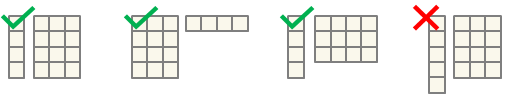Specify two matrices when the coordinates are different among all the plots in both dimensions. Both matrices must have the same size and orientation. The columns of the matrices are plotted against each other.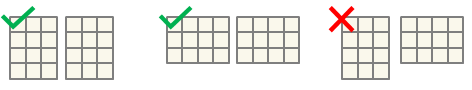## Version History

Introduced before R2006a

expand all